Question

Complex Analysis

When the lighted dot stops, the player wins a certain amount of money if the light is yellow or blue.

The player receives a different amount of money for the colours blue or yellow.

If the lighted dot stops on red, players get their money back.

If the lighted dot stops on green, the player loses their bet.

• The game must be fair.

Determine the amount of money the player receives if the lighted dot stops in a yellow or blue section of the target.Verified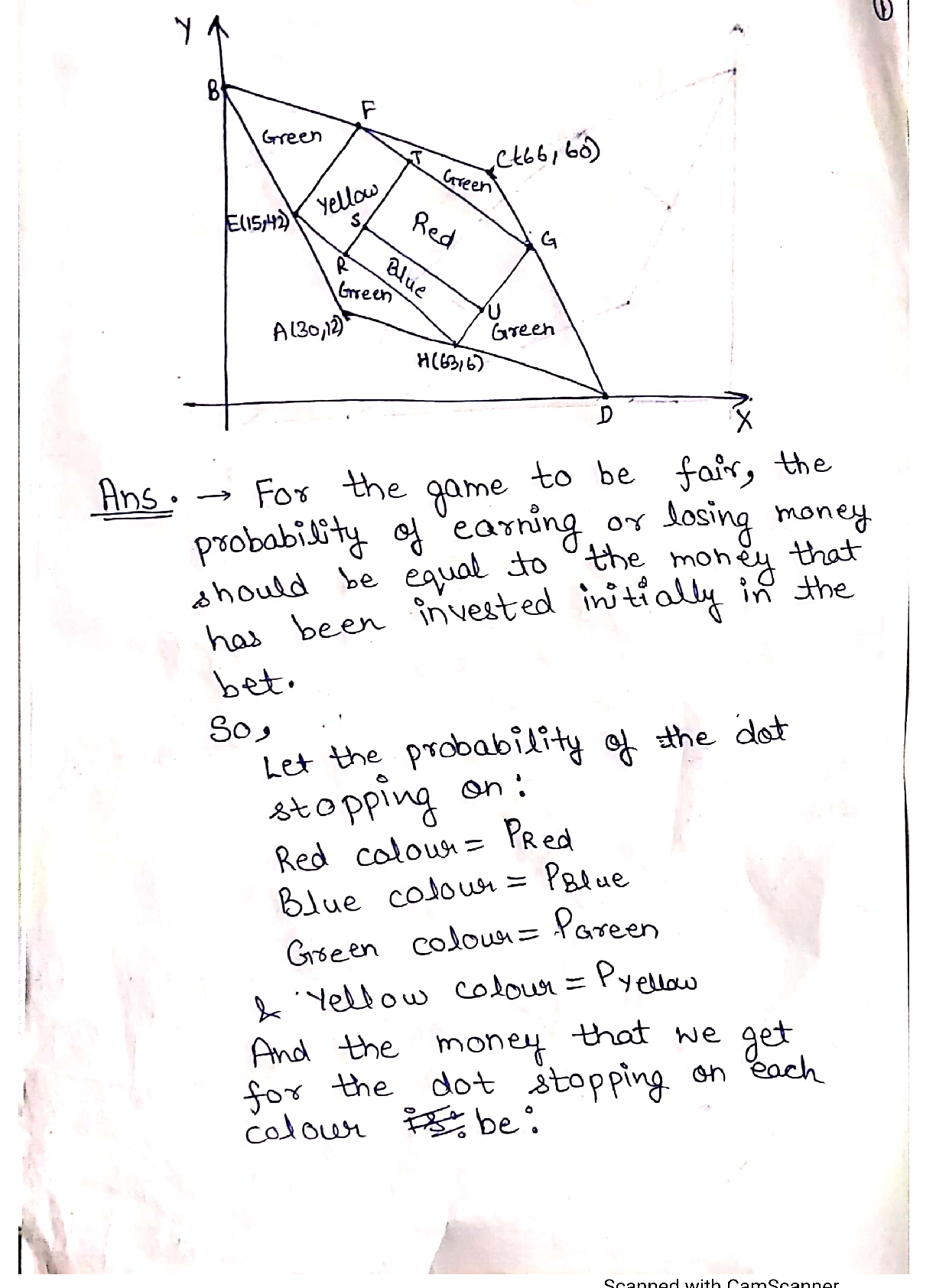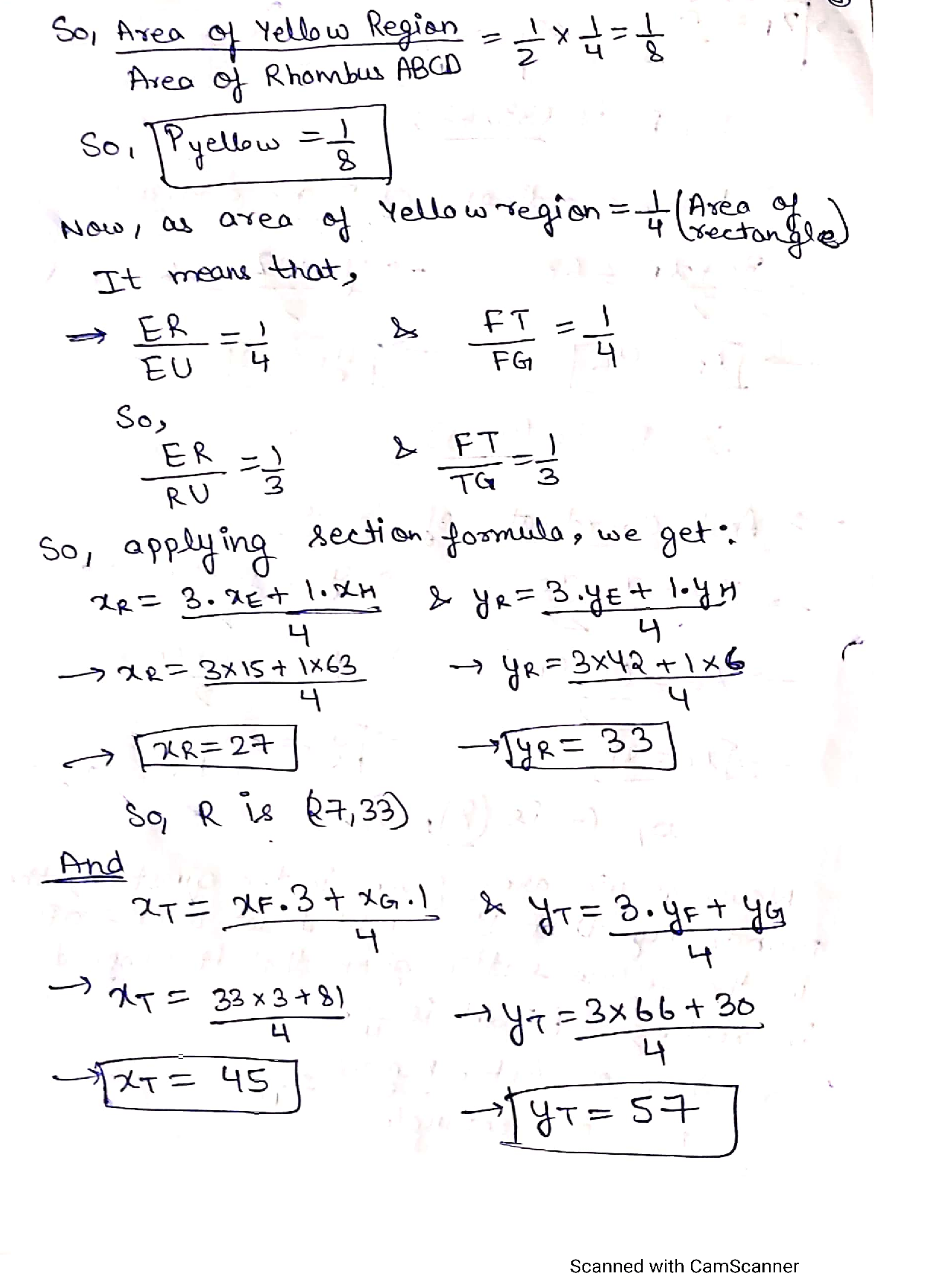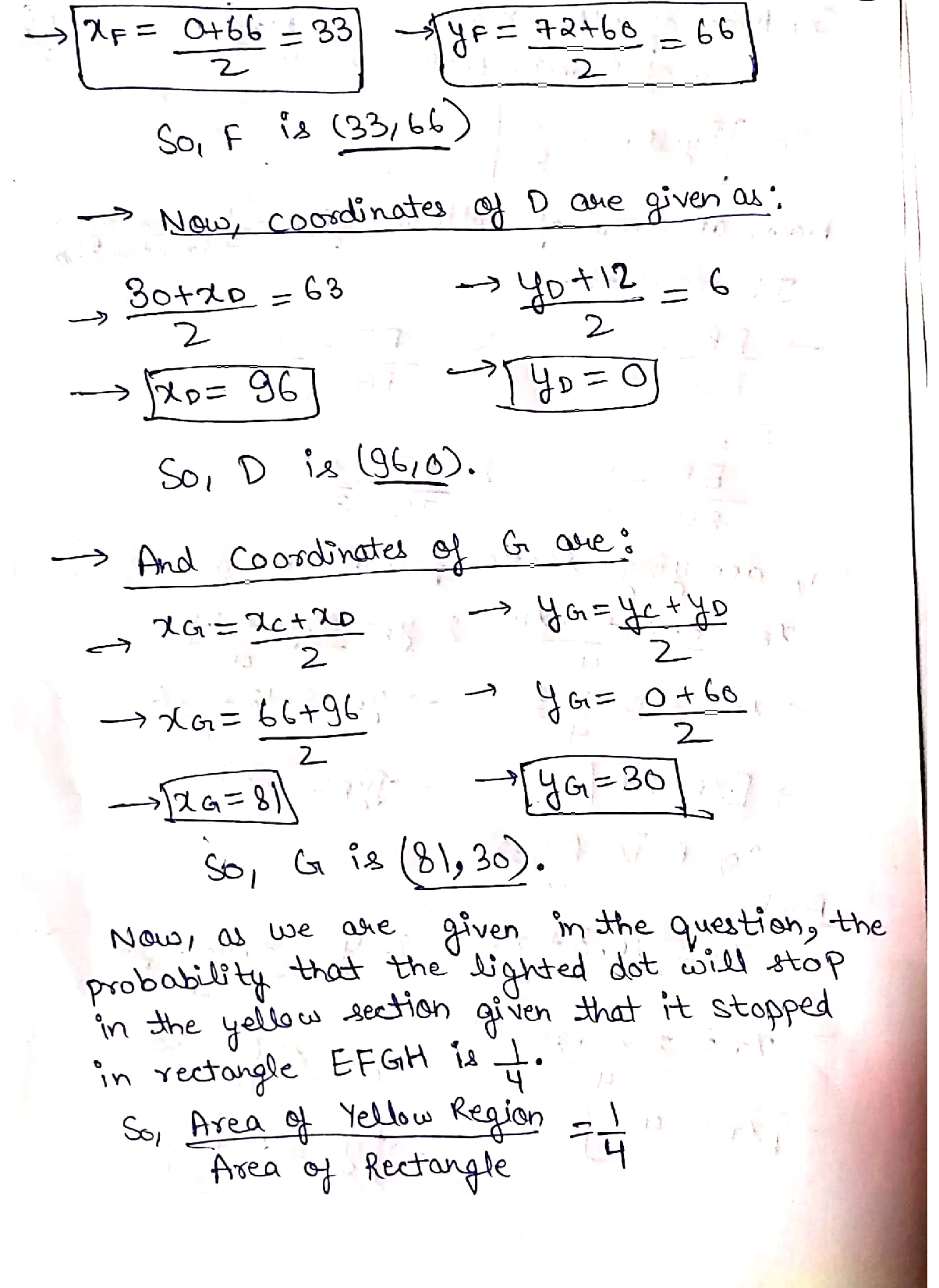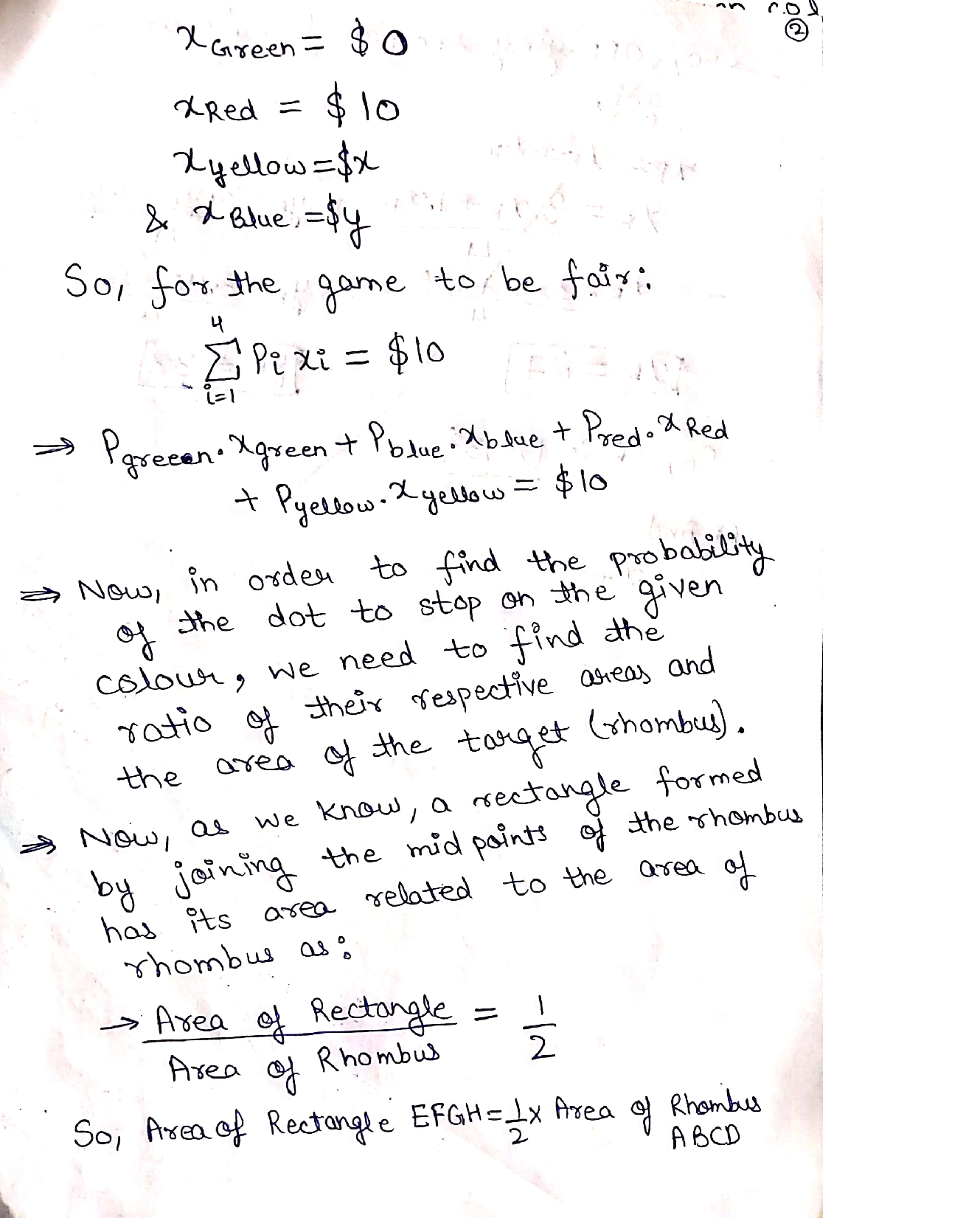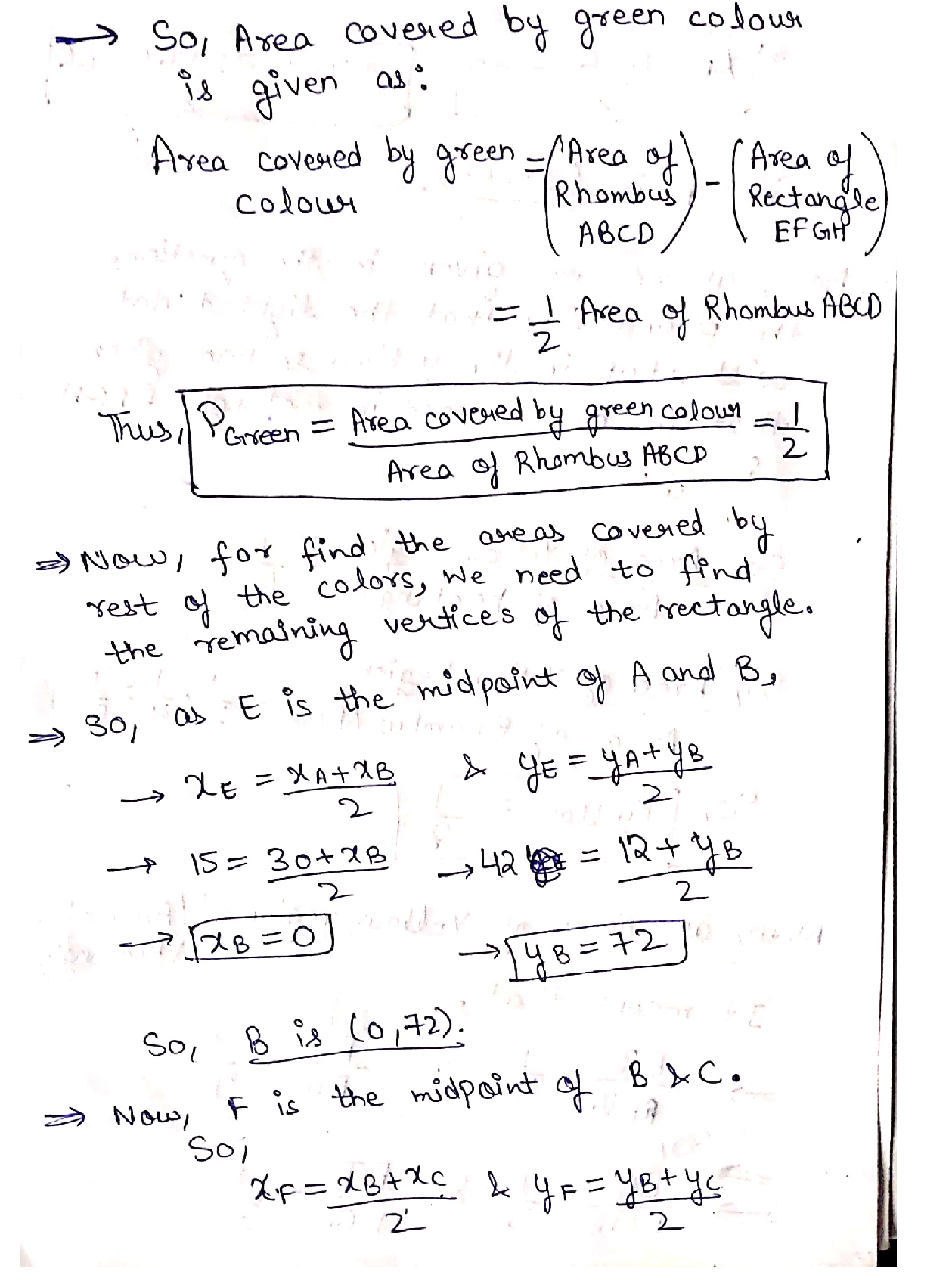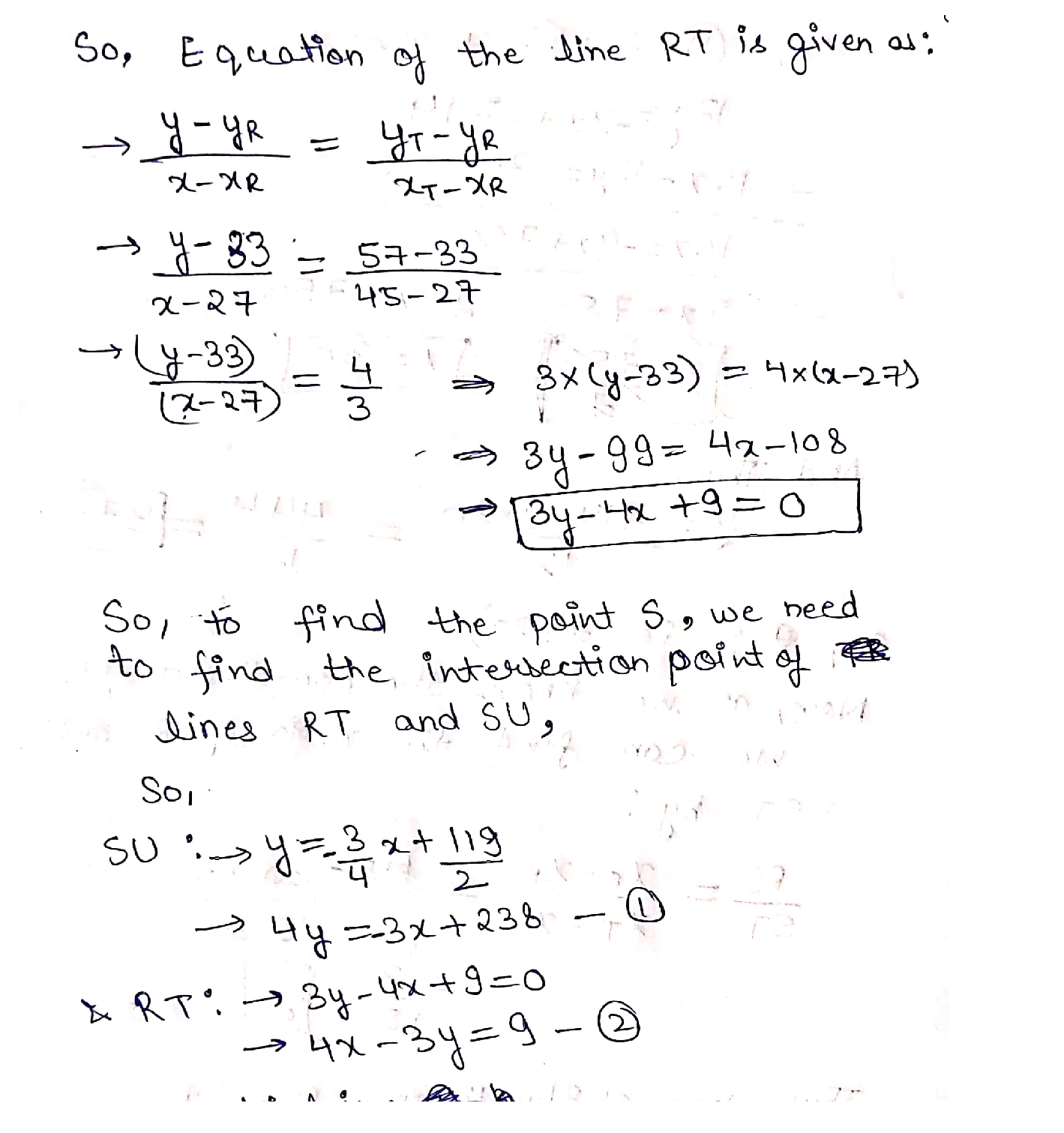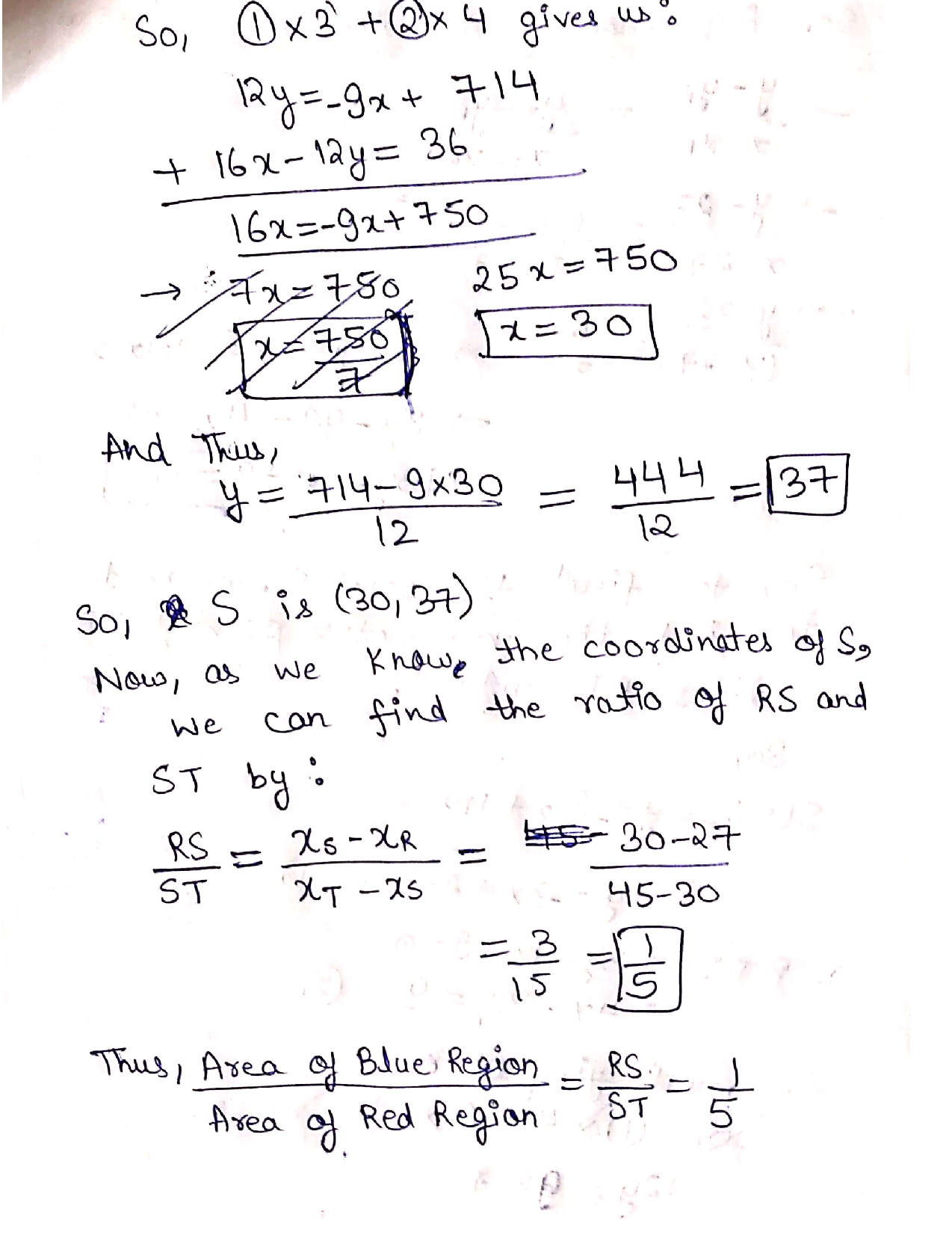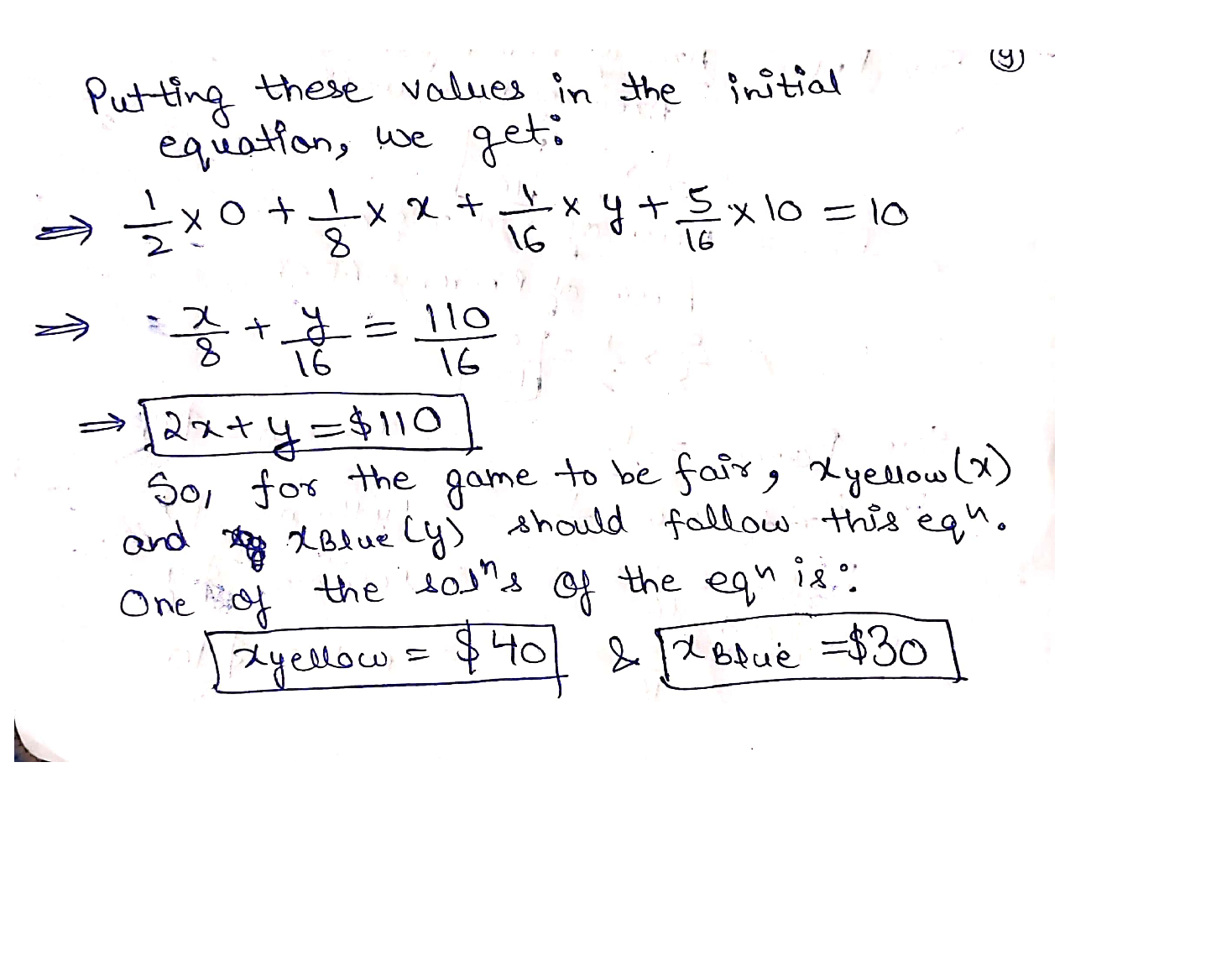### Question 39041Complex Analysis

a) Let C be a set, and A and B be disjoint sets. Find a bijection vh: CA × CB → CAUB¸ and show that it is well-defined, injective,and surjective.
(b) Denote by |X| the cardinality of a set X.
(i) Let A, B, C, and D be sets such that |A| = |B| and |C| =|D]. Prove that
|Ac| = |Bd
(ii) Find a pair of sets A and B so that |A| < |B|, but |AN| =|B]. Find an example where B is countably infinite. (Noproof is necessary.)
(iii) Find two different explicit bijections f, g: 5N → 2N. By explicit, I mean recipes for converting elements of 5N into elements of 2N. Explain your answer graphically in terms of the trees for 2N and 5N.

### Question 29404Complex Analysis

2. If arctan x =y, what is cos y?

### Question 29403Complex Analysis

1. The tide in a river has a 60-cm variation from high tide to low tide. During a 24 hour period, there are two high tides and two low tides. The lowest tide of the river is 11cm and the tidal patterns form a sinusoidal wave. At 1 am, the tide is halfway between high and low tide and dropping.
(a) Sketch a graph of at least one period of this function. Label your graph and define your variables. Let t represent the number of hours since midnight.
(b) Write two different equations that could represent the situation above.
(c) A fisherman prefers to go fishing when the tide is approximately 25cm. Calculate one time when this occurs (give both an exact answer and an answer rounded to two decimal places).

### Question 28538Complex Analysis

(2) Let AABC be an isosceles triangle with AB = AC. Let BM be thealtitude from B on AC and CN be the altitude from C on AB. Show thatBM CN.

### Question 28537Complex Analysis

(1) In a regular polygon, the measure of each interior angle is three times themeasure of the exterior angle. Find the number of sides of this polygon.

### Question 28490Complex Analysis

4. Alex is a furniture designer. He is designing a rectangular desk that must have an area of 7200 cm? and a minimum perimeter,
a. What are the dimensions of the desk, to the nearest tenth of a centimetre?
b. What is the minimum perimeter of the desk?(2 marks)

### Question 28489Complex Analysis

Ainsley has 20 m of fencing which he can use to surround a rectangular garden. What is the maximum area of the garden, assuming Ainsley uses fencing for all four sides?

### Question 28481Complex Analysis

13. An 8 m ladder is leaning against a wall. The foot of the ladder is 6 m from the wall.
a) How far up the wall does the ladder reach? Round your answer to one decimal place. Include a diagram with your solution.(3 marks)
b) What is the angle of elevation of the ladder? Round to the nearest whole number.

### Question 28480Complex Analysis

12. The point R(-5,6) lies on the terminal arm of an angle, 0, in standard position. Determine the primary trigonometric ratios of 0 to four decimal places. (4 marks)

### Question 28479Complex Analysis

11. Elia is installing a new stereo system in her entertainment room. She figures out that the best soundwill occur when the speakers are positioned as shown in the diagram. What is the distance between the two speakers? Round your answer to the nearest tenth. (3 marks)

### Submit query

Getting answers to your urgent problems is simple. Submit your query in the given box and get answers Instantly.

### Submit a new QuerySuccess

Assignment is successfully created